Share

# RD Sharma solutions for Mathematics for Class 9 chapter 23 - Graphical Representation of Statistical Data [Latest edition]

Course
Textbook page

#### Chapters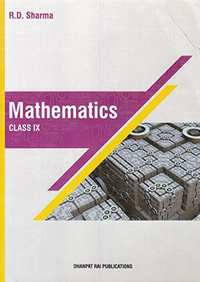## Chapter 23: Graphical Representation of Statistical Data

Ex. 23.1Ex. 23.2Others

#### RD Sharma solutions for Mathematics for Class 9 Chapter 23 Graphical Representation of Statistical DataExercise 23.1 [Pages 7 - 17]

Ex. 23.1 | Q 1 | Page 7

The following table shows the daily production of T. V. sets in an industry for 7 days of a week:

 Day Mon Tue Wed Thurs Fri Sat Sun Number of T.V. Sets 300 400 150 250 100 350 200

Represent the above information by a pictograph .

Ex. 23.1 | Q 2 | Page 7

The following table shows the number of Maruti cars sold by five dealers in a particular month:

 Dealer: Saya Bagga Links D.D. Motors Bhasin Motors Competent Cars sold: 60 40 20 15 10

Represent the above information by a pictograph.

Ex. 23.1 | Q 3 | Page 8

The population of Delhi State in different census years is as given below:

 Census year 1961 1971 1981 1991 2001 Population in Lakhs 30 55 70 110 150
Ex. 23.1 | Q 4 | Page 8

Read the bar graph shown in Fig. 23.8 and answer the following questions: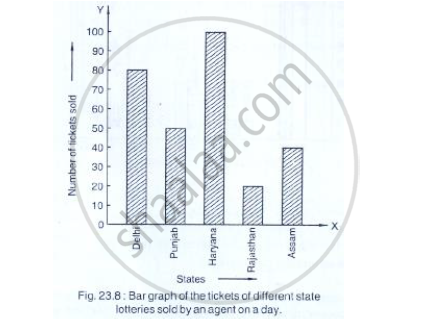(i) What is the information given by the bar graph?
(ii) How many tickets of Assam State Lottery were sold by the agent?
(iii) Of which state, were the maximum number of tickets sold?
(iv) State whether true or false.
The maximum number of tickets sold is three times the minimum number of tickets sold.
(v) Of which state were the minimum number of tickets sold?

Ex. 23.1 | Q 5 | Page 8

Study the bar graph representing the number of persons in various age groups in a town shown in Fig. below. Observe the bar graph and answer the following questions:
(i) What is the percentage of the youngest age-group persons over those in the oldest age group?
(ii) What is the total population of the town?

(iii) What is the number of persons in the age group 60 - 65?
(iv) How many persons are more in the age-group 10 - 15 than in the age group 30 - 35?
(v) What is the age-group of exactly 1200 persons living in the town?
(vi) What is the total number of persons living in the town in the age-group 50 - 55?
(vii) What is the total number of persons living in the town in the age-groups 10 - 15 and 60 - 65?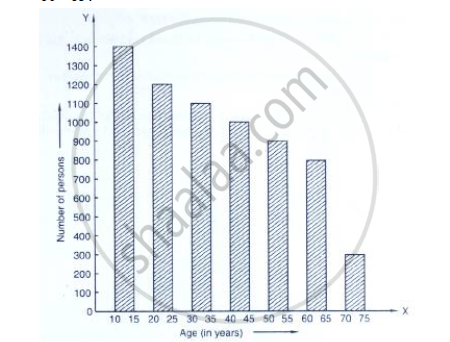(viii) Whether the population in general increases, decreases or remains constant with the increase in the age-group.

Ex. 23.1 | Q 6 | Page 9

Read the bar graph shown in Fig. 23.10 and answer the following questions
(i) What is the information given by the bar graph?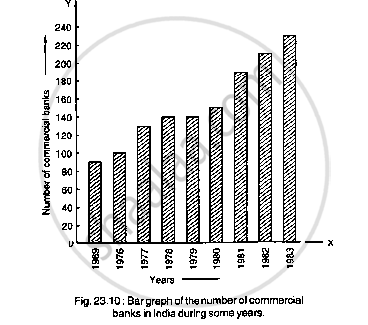(ii) What was the number of commercial banks in 1977?
(iii) What is the ratio of the number of commercial banks in 1969 to that in 1980?
(iv) State whether true or false:
The number of commercial banks in 1983 is less than double the number of commercial banks in 1969.

Ex. 23.1 | Q 7 | Page 10

Given below (Fig. below) is the bar graph indicating the marks obtained out of 50 in mathematics paper by 100 students. Read the bar graph and answer the following questions: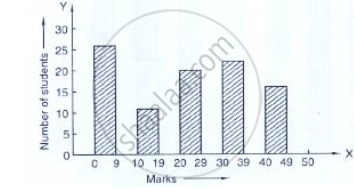(i) It is decided to distribute work books on mathematics to the students obtaining less than 20 marks, giving one workbook to each of such students. If a work book
costs Rs 5, what sum is required to buy the work books?
(ii) Every student belonging to the highest mark group is entitled to get a prize of Rs. 10. How much amount of money is required for distributing the prize money?
(iii) Every student belonging to the lowest mark—group has to solve 5 problems per day. How many problems, in all, will be solved by the students of this group per day?
(iv) State whether true or false.
a. 17% students have obtained marks ranging from 40 to 49.
b. 59 students have obtained marks ranging from 10 to 29.
(v) What is the number of students getting less than 20 marks?
(vi) What is the number of students getting more than 29 marks?
(vii) What is the number of students getting marks between 9 and 40?
(viii) What is the number of students belonging to the highest mark group?
(ix) What is the number of students obtaining more than 19 marks?

Ex. 23.1 | Q 8 | Page 10

Read the following bar graph (Fig. 23.12) and answer the following questions:
(i) What is the information given by the bar graph?
(ii) State each of the following whether true or false.
a. The number of government companies in 1957 is that of 1982 is 1 :9.
b. The number of government companies have decreased over the year 1957 to 1983.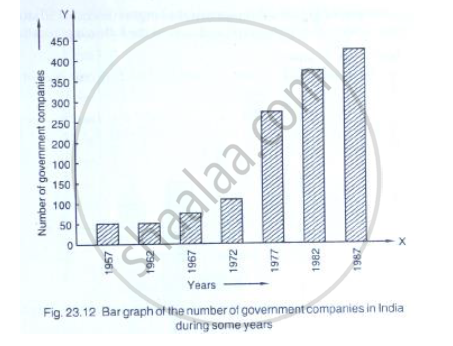Ex. 23.1 | Q 9 | Page 11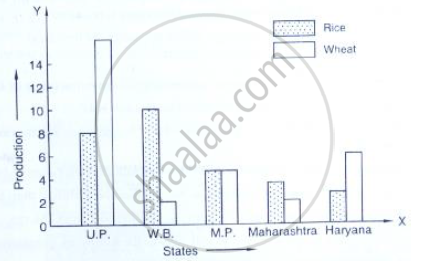(i) What information is given by the bar graph?
(ii) Which state is the largest producer of rice?
(iii) Which state is the largest producer of wheat?
(iv) Which state has total production of rice and wheat as its maximum?
(v) Which state has the total production of wheat and rice minimum?

Ex. 23.1 | Q 10 | Page 12

The following bar graph (Fig. 23. 1 4) represents the heights (in cm) of 50 students of Class XI of a particular school. Study the graph and answer the following questions: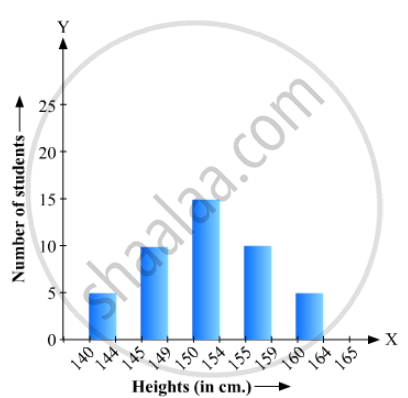(i) What percentage of the total number of students have their heights more than 149cm?
(ii) How many students in the class are in the range of maximum height of the class?
(iii) The school wants to provide a particular type of tonic to each student below the height
of 150 cm to improve his height. If the cost of the tonic for each student comes out to be Rs. 55, how much amount of money is required?
(iv) How many students are in the range of shortest height of the class?
(v) State whether true or false:
a. There are 9 students in the class whose heights are in the range of 155 - 159 cm.
b. Maximum height (in cm) of a student in the class is 17.
c. There are 29 students in the class whose heights are in the range of 145- 154 cm.
d. Minimum height (in cm) of a student is the class is in the range of 140 – 144 cms.
e. The number of students in the class having their heights less than 150 cm is 12.
f. There are 14 students each of whom has height more than 154. cm.

Ex. 23.1 | Q 11 | Page 12

(i) What information is given by the bar graph?
(ii) What was the production of a student in the year 1980 - 81?
(iii) What is the minimum and maximum productions of cement and corresponding years?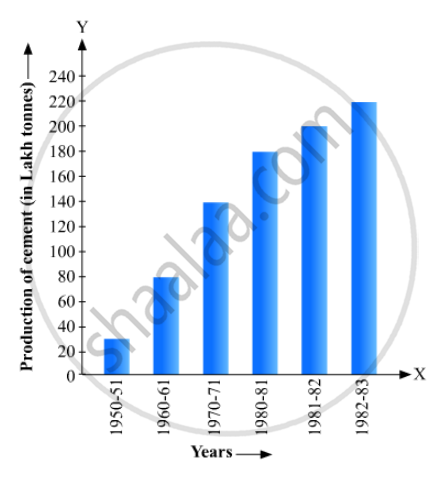Ex. 23.1 | Q 12 | Page 13

The bar graph shown in Fig 23.16 represents the circulation of newspapers in 10 languages. Study the bar graph and answer the following questions: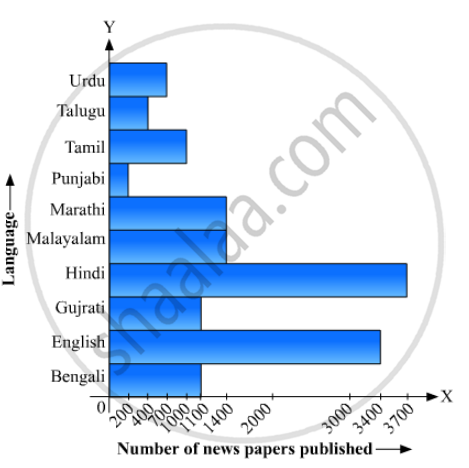(i) What is the total number of newspapers published in Hindi, English, Urdu, Punjabi and Bengali?
(ii) What percent is the number of news papers published in Hindi of the total number of newspapers?
(iii) Find the excess of the number of newspapers published in English over those published in Urdu.
(iv) Name two pairs of languages which publish the same number of newspapers.
(v) State the language in which the smallest number of newspapers are published.
(vi) State the language in which the largest number of newspapers are published.
(vii) State the language in which the number of newspapers published is between 2500 and 3500.
(viii) State whether true or false:
a. The number of newspapers published in Malayalam and Marathi together is less than those published in English.
b. The number of newspapers published in Telugu is more than those published in Tamil.

Ex. 23.1 | Q 13 | Page 14

Read the bar graph given in Fig. 23.17 and answer the following questions:
(i) What information is given by the bar graph?
(ii) What was the crop-production of rice in 1970 - 71?
(iii) What is the difference between the maximum and minimum production of rice?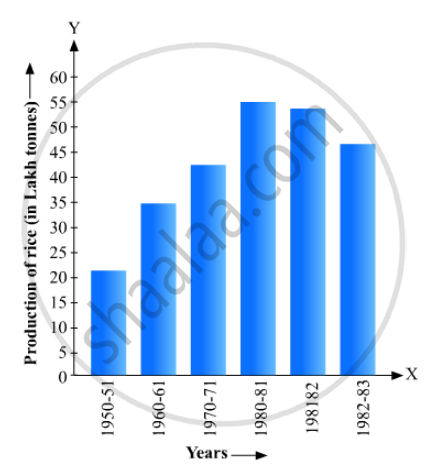Ex. 23.1 | Q 14 | Page 15

Read the bar graph given in Fig. below and answer the following questions: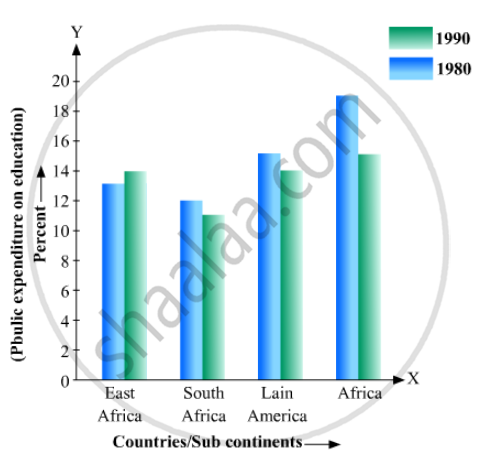(i) What information does it give?
(ii) In which part the expenditure on education is maximum in 1980?
(iii) In which part the expenditure has gone up from 1980 to 1990?
(iv) In which part the gap between 1980 and 1990 is maximum?

Ex. 23.1 | Q 15 | Page 15

Read the bar graph given in Fig. 23.19 and answer the following questions:
(i) What information is given by the bar graph?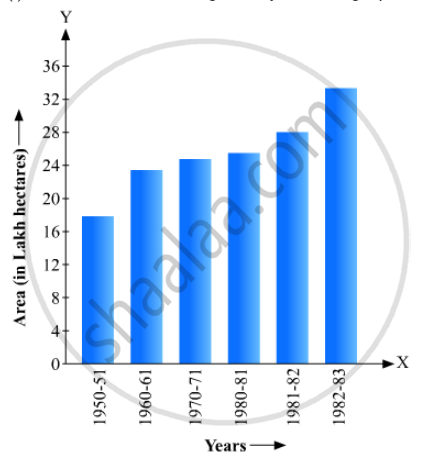(ii) In which years the areas under the sugarcane crop were the maximum and the minimum?
(iii) State whether true or false:
The area under the sugarcane crop in the year 1982 - 83 is three times that of the year 1950 - 51

Ex. 23.1 | Q 16 | Page 16

Read the bar graph given in Fig. 23.20 and answer the fol1owing questions: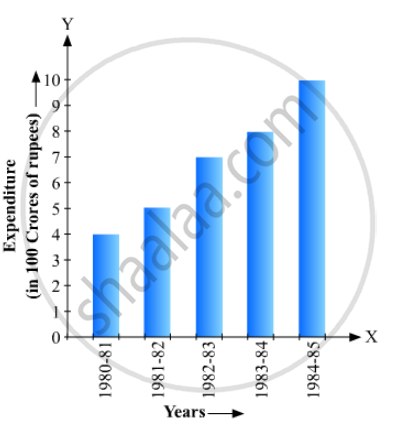(i) What information is given by the bar graph?
(ii) What was the expenditure on health and family planning in the year 1982-83?
(iii) In which year is the increase in expenditure maximum over the expenditure in previous year? What is the maximum increase?

Ex. 23.1 | Q 17 | Page 16

Read the bar graph given in Fig. 23.21 and answer the following questions:
(i) What is the information given by the bar graph?
(ii) What is the number of families having 6 members?
(iii) How many members per family are there in the maximum number of families? Also tell the number of such families.
(iv) What are the number of members per family for which the number of families are equal? Also, tell the number of such families?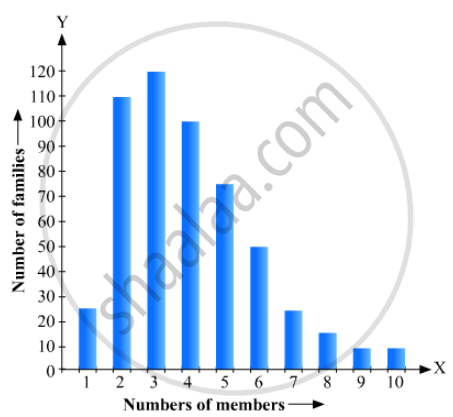Ex. 23.1 | Q 18 | Page 17

Read the bar graph given in Fig. 23.22 and answer the following questions: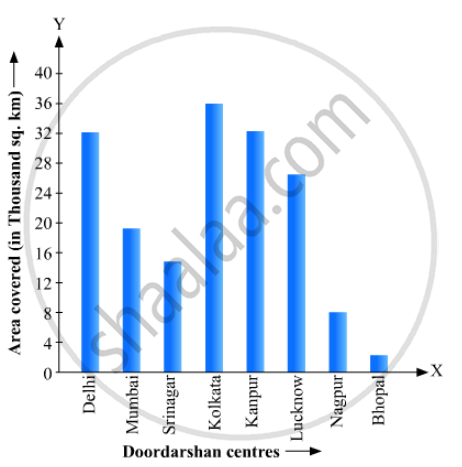(i) What information is given by the bar graph?
(ii) Which Doordarshan centre covers maximum area? Also tell the covered area.
(iii) What is the difference between the areas covered by the centres at delhi and Bombay?
(iv) Which Doordarshan centres are in U.P State? What are the areas covered by them?

#### RD Sharma solutions for Mathematics for Class 9 Chapter 23 Graphical Representation of Statistical DataExercise 23.2 [Pages 23 - 28]

Ex. 23.2 | Q 1 | Page 23

Explain the reading and interpretation of bar graphs.

Ex. 23.2 | Q 2 | Page 23

(i) What information is given by the bar graph?
(ii) In which year the export is minimum?
(iii)In which year the import is maximum?
(iv)In which year the difference of the values of export and import is maximum?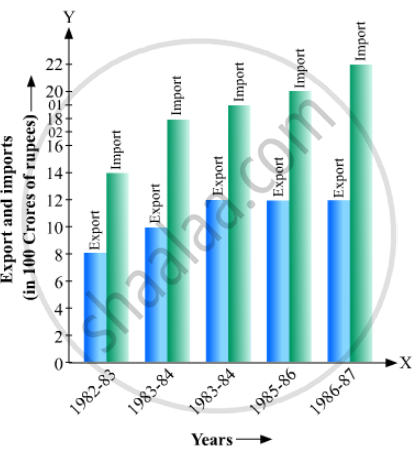Ex. 23.2 | Q 3 | Page 24

The following bar graph shows the results of an annual examination in a secondary school. Read the bar graph  and choose the correct alternative in each of the following: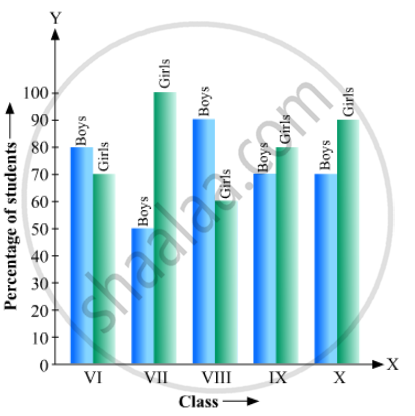(i) The pair of classes in which the results of boys and girls are inversely proportional are:
(a) VI, VIII (b) VI, IX (c) VIII, IX (d) VIII, X
(ii) The class having the lowest failure rate of girls is
(a) VII (b) X (c) IX (d) VIII
(iii)The class having the lowest pass rate of students is
(a) VI (b) VII (c) VIII (d) IX

Ex. 23.2 | Q 4 | Page 25

The following data gives the number (in thousands) of applicants registered with an

 Year 1995 1996 1997 1998 1999 2000 Number of applicants registered (in thousands) 18 20 24 28 30 34

Construct a bar graph to represent the above data.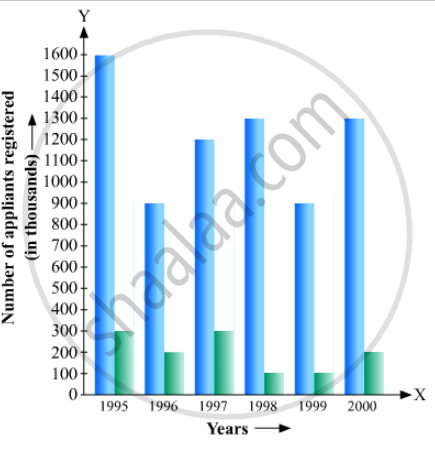Ex. 23.2 | Q 5 | Page 25

The production of saleable steel in some of the steel plants our country during 1999 is given below:

 Plant Bhilai Durgapur Rourkela Bokaro Production(In thousand tonnes) 160 80 200 150

Construct a bar graph to represent the above data on a graph paper by using the scale 1 big divisions = 20 thousand tonnes.

Ex. 23.2 | Q 6 | Page 26

The following table gives the route length (in thousand kilometres) of the Indian Railways in some of the years:

 Year 1960-61 1970-71 1980-81 1990-91 2000-2001 Route length(in thousand km) 56 60 61 74 98

Represent the above data with the help of a bar graph.

Ex. 23.2 | Q 7 | Page 26

The following data gives the amount of loans (in crores of rupees) disbursed by a bank during some years:

 Year 1992 1993 1994 1995 1996 Loan(in crores of rupees) 28 33 55 55 80

(i) Represent the above data with the help of a bar graph.
(ii) With the help of the bar graph, indicate the year in which amount of loan is not increased over that of the preceding year.

Ex. 23.2 | Q 8 | Page 26

The following table shows the interest paid by a company (in lakhs):

 Year 1995-96 1996-97 1997-98 1998-99 1999-2000 Interest (in lakhs of rupees 20 25 15 18 30

Draw the bar graph to represent the above information.

Ex. 23.2 | Q 9 | Page 26

The following data shows the average age of men in various countries in a certain year:

 Country India Nepal China Pakistan U.K U.S.A Average age(in years) 55 52 60 50 70 75

Represent the above information by a bar graph.

Ex. 23.2 | Q 10 | Page 26

The following data gives the production of foodgrains (in thousand tonnes) for some years:

 Year 1995 1996 1997 1998 1999 2000 Production(in thousand tonnes 120 150 140 180 170 190

Represent the above with the help of a bar graph.

Ex. 23.2 | Q 11 | Page 26

The following data gives the amount of manure (in thousand tonnes) manufactured by a company during some years:

 Year 1992 1993 1994 1995 1996 1997 Manure(in thousand tonnes) 15 35 45 30 40 20

(i) Represent the above data with the help of a bar graph.

(ii) Indicate with the help of the bar graph the year in which the amount of manufactured by the company was maximum.

(iii) Choose the correct alternative:
The consecutive years during which there was maximum decrease in manure production are:
(a) 1994 and 1995
(b) 1992 and 1993
(c) 1996 and 1997
(d) 1995 and 1996

Ex. 23.2 | Q 12 | Page 27

The following data gives the demand estimates of the Government of India, Department of Electronics for the personnel in the Computer sector during the Eighth Plan period (1990-95):

 Qualifications: MCA(Master inComputerapplications) DCA(Diploma in Computer Applications) DCE(Diploma in Computer Engineering) CL(Certificate Level Course) ST(Short-term Course) Personnel Required 40600 181600 18600 670600 1802900

Represent the data with the help of a bar graph. Indicate with the help of the bar graph the course where estimated requirement is least.

Ex. 23.2 | Q 13 | Page 27

The income and expenditure for 5 years of a family is given in the following data:

 Years 1995-96 1996-97 1997-98 1998-99 1999-2000 Income(Rs. inthousands) 100 140 150 170 210 Expenditure(Rs. in thousands) 80 130 145 160 190

Represent the above data by a gar graph.

Ex. 23.2 | Q 14 | Page 27

The investment (in ten crores of rupees) of Life Insurance Corporation of India in different sectors are given below:

 Sectors Investment(in ten crores of rupees) Central Government SecuritiesState Government SecuritiesSecurities guaranteed by the GovernmentPrivate SectorsSocially oriented sectors (Plans)Socially oriented sectors (Non-Plan) 451123184611

Represent the above data with the help of bar graph.

Ex. 23.2 | Q 15 | Page 27

The following data gives the value (in crores of rupees) of the Indian export of cotton textiles for different years:

 Years 1982 1983-1984 1984-1985 1985-1986 1986-1987 Value of Exportof Cotton Textiles(in crores of rupees) 300 325 475 450 550

Represent the above data with the help of a bar graph. Indicate with the help of a bar graph the year in which the rate of increase in exports is maximum over the preceding year.

Ex. 23.2 | Q 16 | Page 28

The following tables gives the quantity of goods (in crore tonnes)

 Year 1950-51 1960-61 1965-66 1970-71 1980-81 1982-83 Quantity of Goods(in crore tonnes) 9 16 20 20 22 26
Represent this information with the help of a bar graph.
Explain through the bar graph if the quantity of goods carried by the Indian Railways in 1965-66 is more than double the quantity of goods carried in the year 1950-51.
Ex. 23.2 | Q 17 | Page 28

The production of oil (in lakh tonnes) in some of the refineries in India during 1982 was given below:

 Refinery: Barauni Koyali Mathura Mumbai Florida Production of oil(in lakh tonnes) 30 70 40 45 25

Construct a bar graph to represent the above data so that the bars are drawn horizontally.

Ex. 23.2 | Q 18 | Page 28

The expenditure (in 10 crores of rupees) on health by the Government of India during the various five year plans is shown below:

 Plans: I II III IV V VI Expenditure on health(in 10 crores of rupees) 7 14 23 34 76 182

Construct a bar graph to represent the above data.

#### RD Sharma solutions for Mathematics for Class 9 Chapter 23 Graphical Representation of Statistical Data [Pages 41 - 42]

Q 1 | Page 41

Construct a histogram for the following data:

 Monthly Schoolfee (in Rs): 30-60 60-90 90-120 120-150 150-180 180-210 210-240 No of Schools 5 12 14 18 10 9 4
Q 2 | Page 41

The distribution of heights (in cm) of 96 children is given below. Construct a histogram and a frequency polygon on the same axes.

 Height (in cm): 124to128 128to132 132to136 136to140 140to144 144to148 148to152 152to156 156to160 160to164 No. of Children: 5 8 17 24 16 12 6 4 3 1
Q 3 | Page 41

The time taken, in seconds, to solve a problem by each of 25 pupils is as follows:
16, 20, 26, 27, 28, 30, 33, 37, 38, 40, 42, 43, 46, 46, 46, 48, 49, 50, 53, 58, 59, 60, 64, 52, 20
(a) Construct a frequency distribution for these data, using a class interval of 10 seconds.
(b) Draw a histogram to represent the frequency distribution.

Q 4 | Page 41

Draw, in the same diagram, a histogram and a frequency polygon to represent the following data which shows the monthly cost of living index of a city in a period of 2 years:

 Cost of livingindex: 440-460 460-480 480-500 500-520 520-540 540-560 560-580 580-600 No. of months: 2 4 3 5 3 2 1 4
Q 5 | Page 42

The following is the distribution of total household expenditure (in Rs.) of manual worker in a city:

 Expenditure(in Rs): 100-150 150-200 200-250 250-300 300-350 350-400 400-450 450-500 No. of manual workers: 25 40 33 28 30 22 16 8

Draw a histogram and a frequency polygon representing the above data.

Q 6 | Page 42

The following table gives the distribution of IQ's (intelligence quotients) of 60 pupils of class V in a school:

 IQ's: 125.5to13.25 118.5to125.5 111.5to118.5 104.5to111.5 97.5to104.5 90.5to97.5 83.5to90.5 76.5to83.5 69.5to76.5 62.5to69.5 No.of pupils: 1 3 4 6 10 12 15 5 3 1

Draw a frequency polygon for the above data.

Q 7 | Page 42

Draw a histogram for the daily earnings of 30 drug stores in the following table:

 Daily earning(in Rs): 450-500 500-550 550-600 600-650 650-700 Number of Stores: 16 10 7 3 1
Q 8 | Page 42

The monthly profits (in Rs.) of 100 shops are distributed as follows:

 Profits per shop: 0-50 50-100 100-50 150-200 200-250 250-300 No. shops: 12 18 27 20 17 6

Draw a histogram for the data and show the frequency polygon for it.

#### RD Sharma solutions for Mathematics for Class 9 Chapter 23 Graphical Representation of Statistical Data [Pages 42 - 43]

Q 1 | Page 42

Which one of the following is not the graphical representation of statistical data:

•  Bar graph

• Histogram

• Frequency polygon

• Cumulative frequency distribution

Q 2 | Page 42

In a frequency distribution, ogives are graphical representation of

• Frequency

•  Relative frequency

• Cumulative frequency

•  Raw data

Q 3 | Page 42

A frequency polygon is constructed by plotting frequency of the class interval and the

• upper limit of the class

•  lower limit of the class

• mid value of the class

•  any values of the class

Q 4 | Page 43

In a histogram the area of each rectangle is proportional to

•  the class mark of the corresponding class interval

• the class size of the corresponding class interval

• frequency of the corresponding class interval

• cumulative frequency of the corresponding class interval

Q 5 | Page 43

In the 'less than' type of ogive the cumulative frequency is plotted against

•  the lower limit of the concerned class interval

•  the upper limit of the concerned class interval

•  the mid-value of the concerned class interval

• any value of the concerned class interval

Q 6 | Page 43

In a histogram the class intervals or the group are taken along

•  Y-axis

• X-axis

• both of X-axis and Y-axis

•  in between X and Y axis

Q 7 | Page 43

A histogram is a pictorial representation of the grouped data in which class intervals and frequency are respectively taken along

•  vertical axis and horizontal axis

• vertical axis only

•  horizontal axis only

•  Horizontal axis and vertical axis

Q 8 | Page 43

In a histogram, each class rectangle is constructed with base as

• frequency

• class-intervals

• range

• size of the class

## Chapter 23: Graphical Representation of Statistical Data

Ex. 23.1Ex. 23.2Others## RD Sharma solutions for Mathematics for Class 9 chapter 23 - Graphical Representation of Statistical Data

RD Sharma solutions for Mathematics for Class 9 chapter 23 (Graphical Representation of Statistical Data) include all questions with solution and detail explanation. This will clear students doubts about any question and improve application skills while preparing for board exams. The detailed, step-by-step solutions will help you understand the concepts better and clear your confusions, if any. Shaalaa.com has the CBSE Mathematics for Class 9 solutions in a manner that help students grasp basic concepts better and faster.

Further, we at Shaalaa.com provide such solutions so that students can prepare for written exams. RD Sharma textbook solutions can be a core help for self-study and acts as a perfect self-help guidance for students.

Concepts covered in Mathematics for Class 9 chapter 23 Graphical Representation of Statistical Data are Introduction of Statistics, Collection of Data, Presentation of Data, Graphical Representation of Data, Measures of Central Tendency.

Using RD Sharma Class 9 solutions Graphical Representation of Statistical Data exercise by students are an easy way to prepare for the exams, as they involve solutions arranged chapter-wise also page wise. The questions involved in RD Sharma Solutions are important questions that can be asked in the final exam. Maximum students of CBSE Class 9 prefer RD Sharma Textbook Solutions to score more in exam.

Get the free view of chapter 23 Graphical Representation of Statistical Data Class 9 extra questions for Mathematics for Class 9 and can use Shaalaa.com to keep it handy for your exam preparation

S